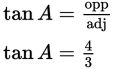# SAT Math Multiple Choice Question 995: Answer and Explanation

### Test Information

Question: 995

15.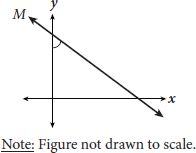The equation of line M shown above is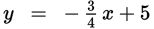. Given that angle A is the acute angle formed by the intersection of line M and the y-axis, which expression could be used to find the measure of angle A?

• A.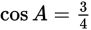• B.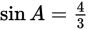• C.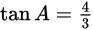• D.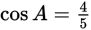Explanation:

C

Difficulty: Hard

Category: Additional Topics in Math / Trigonometry

Strategic Advice: The answer choices are all trig expressions, so use SOH CAH TOA and your equation solving skills.

Getting to the Answer: To find an angle in a triangle using trig, you need to know the measure of another angle and a side length, or two side lengths. It doesn't appear that you have any of these here. However, you do know the slope of the line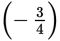, which you can use to sketch in a triangle that contains angle A. Your sketch might look like the following: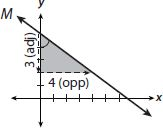You now know the lengths of the sides that are opposite and adjacent to angle A. This means you can use tangent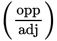to represent the measure of the angle. (Don't worry about whether 3 is positive or negative-you are using the length of the side, which is positive.)